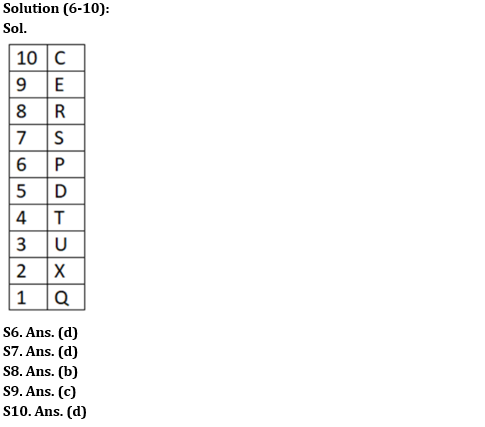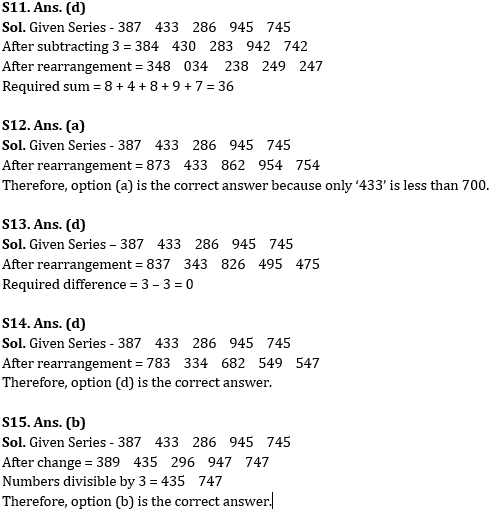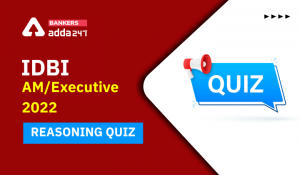Latest Banking jobs   »

# Reasoning Ability Quiz For SBI Clerk Prelims 2022- 1st June

Directions (1-5): Study the following information carefully and answer the given questions.
Nine boxes i.e., P, Q, R, S, T, U, V, W, and X are kept one above the other in a stack. The lowermost stack is numbered 1, the stack above it is numbered 2, and so on until the topmost stack is numbered 9. All the information is not necessarily in the same order.
Three boxes are placed between box P and box X. Two boxes are placed between Box X and Box Q. Box W is placed four places above Box Q. Four boxes are placed between Box W and Box R. Two boxes are placed between Box R and Box S. Box U is placed adjacent to Box S. Box T is placed below Box V but not immediate below. Box V does not place at the top.

Reasoning Ability Quiz For SBI Clerk Prelims 2022 1st June CHECK IN HINDI

Q1. How many boxes are placed between Box U and Box P?
(a) Three
(b) One
(c) Two
(d) None
(e) More than three

Q2. Which amongst the following box is placed three places below Box R?
(a) Box T
(b) Box X
(c) Box V
(d) Box U
(e) None of these

Q3. What is the position of Box Q from top?
(a) Second
(b) Third
(c) Fifth
(d) Seventh
(e) Fourth

Q4. How many boxes are placed below box P?
(a) Five
(b) Three
(c) One
(d) Six
(e) Two

Q5. Which amongst the following box is placed on topmost position?
(a) U
(b) W
(c) P
(d) S
(e) None of these

Directions (6-10): Study the following information carefully and answer the questions accordingly.
Ten people live in a ten-story building. The ground floor is numbered as 1, above it is 2 and so on till the top floor is numbered as 10. T lives on the fourth floor. Two people live between S and T. Four people live between X and S. Q lives immediately below X. Four people live between Q and P. R lives two floors above P. C lives immediately above E. U lives below D.

Q6. Who lives on the second floor?
(a) Q
(b) D
(c) P
(d) X
(e) None of these

Q7. Who lives between C and R?
(a) P
(b) T
(c) S
(d) E
(e) None of these

Q8. Who lives on the fifth floor?
(a) P
(b) D
(c) U
(d) S
(e) None of these

Q9. How many persons live above T?
(a) Two
(b) Four
(c) Six
(d) Five
(e) None of these

Q10. Four among the following five are same in a certain manner and forms a group. Which among the following does not belong to the group?
(a) U, Q
(b) R, C
(c) T, P
(d) S, C
(e) S, D

Directions (11-15): Study the following information carefully and answer the questions accordingly.

387   433   286   945   745

Q11. If 3 is subtracted from each of the numbers and then the digits in all numbers are arranged in ascending order within the number then what will be the sum of the last digits of all the numbers thus formed?
(a) 34
(b) 41
(c) 45
(d) 36
(e) None of these

Q12. If the digits in all the numbers are arranged in descending order within the number, then how many numbers thus formed are less than 700?
(a) One
(b) Three
(c) Two
(d) Four
(e) None of these

Q13. If the first and the second digits of all the numbers are interchanged then what is the difference between the second digit of largest number and the third digit of the smallest number thus formed?
(a) 1
(b) 6
(c) 5
(d) 0
(e) None of these

Q14. If the first and the third digits of all the numbers are interchanged then which of the following numbers will be the smallest?
(a) 387
(b) 745
(c) 286
(d) 433
(e) None of these

Q15. If the second digit of all the even numbers is increased by 1 and the third digit of all the odd numbers is increased by 2 then how many numbers thus formed will be divisible by 3?
(a) One
(b) Two
(c) Four
(d) Three
(e) None

Solutions#### Congratulations!Incorrect details? Fill the form again here

•Reasoning Ability Quiz For IDBI AM/Execu...
•Reasoning Ability Quiz For IBPS RRB PO P...
•Reasoning Ability Quiz For IBPS RRB PO P...
•Reasoning Ability Quiz For IBPS RRB PO P...
•Reasoning Ability Quiz For IBPS RRB PO P...
•Reasoning Ability Quiz For IDBI 2022- 2n...